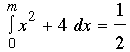SEARCH HOMEMath Central Quandaries & QueriesQuestion from amper, a student: How could i get the median with this equation f(x)=(x^2+4)3/13 0Amper,

The median is the number m such that. This results in a cubic equation which you can solve using Wolfram Alpha.

HarleyMath Central is supported by the University of Regina and The Pacific Institute for the Mathematical Sciences.